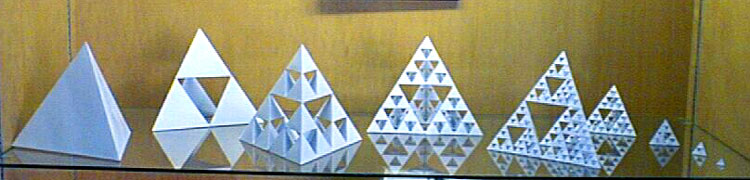# Many, many octahedra

This shelf is displaying several Sierpinski tetrahedra:The linear dimensions of the five big ones from left to right are 1, 2, 4, 8, and 16.

You can fill the void in the second one perfectly with one octahedron.
The third one can be filled with five octahedra, a big one and four smaller ones.

How many octahedra (of different sizes) would it take to fill all the voids in a Sierpinski tetrahedra of linear dimension 1024?

Image credit: http://www.fractalnature.com/

×